Next: 3.4 Digit classification Up: 3.3 Extension of the Previous: 3.3.1 Algorithm

## 3.3.2 Simulations

The simulations show the operation of MPPCA-ext on synthetic distributions. The examples demonstrate the importance of the initialization, the occurrence of empty units, and that the algorithm can separate overlapping ellipses. Finally, some tests compare MPPCA-ext with NGPCA. As in section 3.2.3 the ring-line-square sets with 850 points and with 85 points were used. Further pattern sets are a three-dimensional spiral distribution and a two-dimensional two-lines distribution. The spiral is composed of 1000 points, its radius is 1.2, and its length is 5.0. The points were uniformly distributed along the spiral, which had a thickness of 0.02. The two-lines distribution contained two slightly tilted lines (length: 5.1, thickness: 0.2), each of them consisted of 50 points.

The number of units used was ten for all distributions but two-lines, for which two units were used. For all tests, two principal components were extracted. Both Neural Gas for the initialization and NGPCA used the same parameter set as in section 3.2.3. For MPPCA-ext, the number of expectation and maximization steps is either given or the algorithm iterates until convergence. In all tests, the log-likelihood per pattern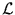was evaluated (see section 3.2.3).

Using the ring-line-square distribution with 850 points, the first test shows the importance of a good initialization. Here, the other modifications did not matter. Figure 3.4 shows that the initialization of the center positions with k-means may lead to undesired local maxima. On the other hand, the Neural Gas initialization reliably resulted in good model fits (figure 3.5). Over ten separate training cycles, the log-likelihood ranged between -1.663 and -1.653. Figure 3.5 further demonstrates how the ellipses move to fit the distribution better.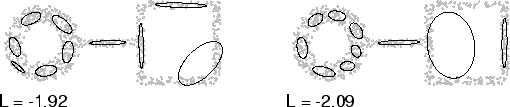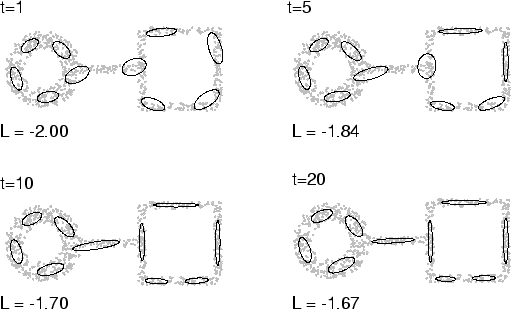The second test on the same distribution demonstrates that the ellipses can spread meaningful over the distribution after they all overlap at the beginning (figure 3.6)3.1. A PCA extracted the two eigenvectors and the corresponding eigenvalues of the covariance matrix of the pattern set. All ten units started with these eigenvectors and eigenvalues; their centers were distributed around the center of the distribution, with random deviations along the principal component. Prior and posterior probabilities were initially the same for all units. Here, the initialization with a single PCA led to a good model fit. However, this does not work for all distributions; therefore, Neural Gas was used instead. Neural Gas also results in a faster convergence (compare the t values between figure 3.5 and figure 3.6).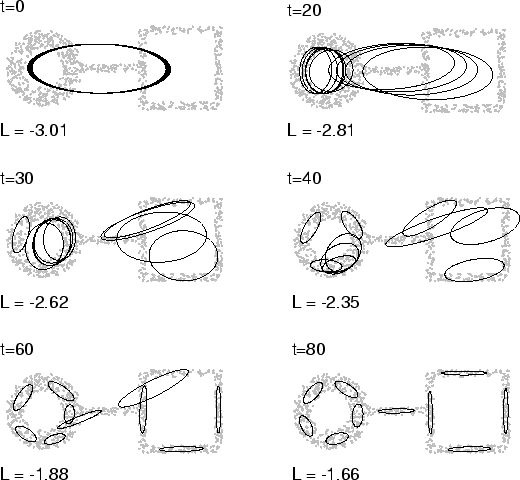Using the sparse ring-line-square set (85 points), the third test shows the occurrence of an empty unit and the consequences of the following correction. Empty units were only observed for sparse distributions. Figure 3.7 illustrates the removal and reappearance of an empty unit. This figure and figure 3.3 already show a comparison between MPPCA-ext, NGPCA, and NGPCA-constV. Before the empty unit correction, the fitted models of MPPCA-ext and NGPCA-constV resembled each other.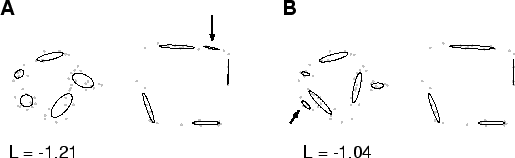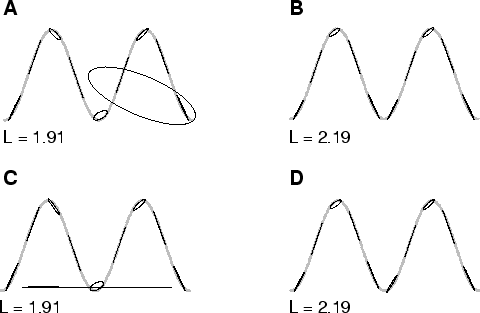The thin spiral and the two-lines distributions were used for more comparisons between the different algorithms. Figure 3.8.A shows that NGPCA ends up with a dead ellipsoid on the thin spiral. However, the dead ellipsoid can be avoided if the annealing is slower ( tmax = 300 000) (figure 3.8.B). In contrast, NGPCA-constV for slow and fast annealing produces an undesired long ellipsoid that stretches to distant parts of the distribution (figure 3.8.C). Like the slow NGPCA, MPPCA-ext produces a good fitted model (figure 3.8.D). The next test shows an example on which MPPCA-ext failed. Using the two-lines distribution, the expectation-maximization iteration ends in an inappropriate local maximum because the Neural Gas initialization cannot distinguish between the two lines (figure 3.9.A). In contrast, both NGPCA variants can cope with the two-lines distribution (figure 3.9.B).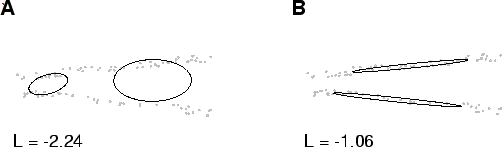Next: 3.4 Digit classification Up: 3.3 Extension of the Previous: 3.3.1 Algorithm
Heiko Hoffmann
2005-03-22